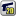# ForumCS2DScriptsstring.len(Bad Argument?)

#string.len(Bad Argument?)

6 replies
Goto Page1Ranw-X
User
OfflineI used string.len in my script, but cs2d couldn't find it and it said "ERROR" "LUA ERROR: sys/lua/******: bad argument #1 to 'len' (string expected, got nil)What is the problem?
edited 1×, last 02.01.19 04:08:23 pm
VTA Community Discord: https://discord.gg/6rHKWvJ
Fraizeraust
Moderator
OfflineCare to show the code?
Ranw-X
User
OfflineCode:
1
2
3
4
5
6
7
8
9
10
11
12
13
function Color(r,g,b)
if not r then r = 0 end
if not g then g = 0 end
if not b then b = 0 end
local r,g,b = tostring(r),tostring(g),tostring(b)
while string.len(r) < 3 do r = "0"..r end
while string.len(g) < 3 do g = "0"..g end
while string.len(b) < 3 do b = "0"..b end
if string.len(r) == 3 and string.len(g) == 3 and string.len(b) == 3 then
return "\169"..r..g..b
end
return "\169255255255"
end
VTA Community Discord: https://discord.gg/6rHKWvJ
deletemyacc
User
Offlineedited 1×, last 28.01.19 04:57:59 pm
DC
OfflineYou left out important info: The line number (the part behind the ":"). Knowing the line would make it much easier to identify the problem.

The function runs fine in the Lua demo web console https://www.lua.org/cgi-bin/demo
print(Color(1,2,3))
line for testing, even
print(Color())
works without error)

Are you sure that the error occurs in the snippet you showed here?

btw: The "local"-statement is misleading/useless. r, g & b are already local variables because they are passed in as function parameters. Therefore you can remove the "local"-statement (in more strict languages keeping it there would lead to an error).
www.UnrealSoftware.de | www.CS2D.com | www.CarnageContest.com | Use the forum & avoid PMs!
Cure Pikachu
User
OfflineThe code provided works OK as it is, at least on my game session.Vehk
User
OfflineYou're probably passing a bad value to the color function (although tostring should always return a string), or the problem is elsewhere in your code.

Show us the entire error message and script. I do not thing the error is raised here.

It looks like there is nothing that can be passed to tostring that would result in it returning nil, so the error must be raised somewhere else.

Code:
1
2
3
4
if string.len(r) == 3 and string.len(g) == 3 and string.len(b) == 3 then
return "\169"..r..g..b
end
return "\169255255255"

The second return statement is never reached because you've made sure that r, g, and b are length 3. So theres no need for it.

Code:
1
local r,g,b = tostring(r),tostring(g),tostring(b)

As DC said this is redundant. And I'd like to point out that there is no need to use tostring here, the numbers will be automatically coerced into strings as needed.

Code:
1
2
3
4
5
6
while string.len(r) < 3 do r = "0"..r end
while string.len(g) < 3 do g = "0"..g end
while string.len(b) < 3 do b = "0"..b end
if string.len(r) == 3 and string.len(g) == 3 and string.len(b) == 3 then
return "\169"..r..g..b
end

This is a lot of work. Check out string.format, it'll simplify this.

Here's a simplified version of this function using string.format
Code:
1
2
3
function Color(r, g, b)
return string.format("\169%0.3u%0.3u%0.3u", r or 0, g or 0, b or 0)
end
edited 1×, last 06.01.19 01:07:08 am1﻿